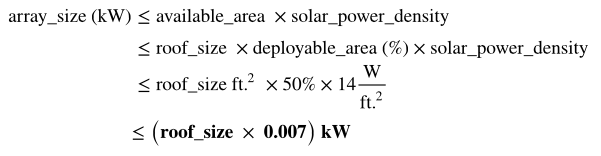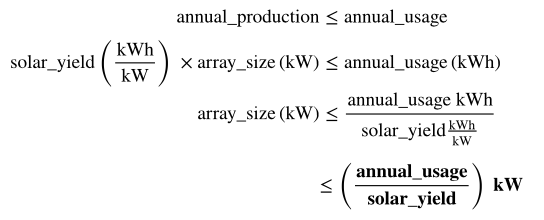Station A Knowledge Base

# How does Station A calculate the size of a solar system for a given building?

## In sizing solar systems, we calculate two potential limits on the size of a solar array at this building.

We use the smaller of the two as the technical potential:

• The capacity that can fit on the roof of the building, accounting for 70% obstruction, a 4ft installation setback, and the typical multi-crystalline silicon solar module installation power density of 14W per sq. ft.
• The maximum capacity that will not make the customer a net exporter of energy; doing so would prevent the customer from being fully compensated under "net metering" or "net billing" rules. We might reduce the size we recommend to maximize the financial return measured in Net Present Value (NPV) to account for economic factors such as net metering or billing.

The following are the calculations behind the roof capacity and net metering limits that we use:

• For roof capacity:• For net-metering limits: